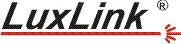Fiber Optic Communications Systems

## What are the limitations of optical fiber?

There are two basic limitations that an optical fiber has. The first is actual loss of light as it travels through the fiber and the other is a maximum limitation of the bandwidth of the signals that can be carried. Loss of light in an optical fiber is the result of absorption and impurities within the glass itself as well as losses caused by mechanical strains that bend the fiber at an angle that is so sharp that the light is actually able to "leak out" through the cladding region. Losses are also dependent on the wavelength of the light employed since the amount of light absorbed by glass varies at different wavelengths. At 850 nanometers, the wavelength most commonly used in short-range transmission systems, typical fiber has a loss of 4 to 5 dB per kilometer of length. At 1310 nanometers this loss drops to under 3 dB per kilometer and at 1550 nanometers, the loss is a dB or so. The last two wavelengths are therefore obviously used for longer transmission distances. As a point of reference, typical well designed fiber optic transmission systems can sustain losses of anywhere from 10 to 30dB.

Losses due to attenuation are independent of the frequency or data rate of the signals being transmitted. There is another loss factor however that is frequency related and is due to the fact that light can have many paths through a fiber. Figure 5 shows the mechanism of this loss through step-index fiber.A light path nearly straight through a fiber is shorter than a light path with maximum "bouncing." This means that for a fast rise-time pulse of light at the input to the fiber, some paths will result in light reaching the end of the fiber sooner than through other paths. This causes a smearing or spreading effect on the output rise (and fall) time of the light pulse which limits the maximum speed of light changes that the fiber will tolerate. Since data is usually transmitted by pulses of light, this, in essence, limits the maximum data rate of the fiber. The spreading effect for a fiber usually is expressed in terms of MHz per kilometer. Standard 62.5 micron core multimode fiber has a typical bandwidth limitation of 160 MHz per kilometer at 850 nanometers and 500 MHz per kilometer at 1310 nanometers. This is due to its large core size relative to the wavelength of the propagated light. Single mode fiber on the other hand, because of its very small 8 micron core diameter has a bandwidth of thousands of MHz per kilometer at 1310 nanometers. For most low frequency applications however, the loss of light due to absorption will limit the transmission distance rather than the pulse spreading effect.

 LuxLink Voice: 1-516-931-2800 Email: